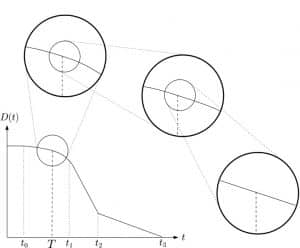# Differentiation – finding the gradient of curves

## Differentiation

Differentiation (which, like integration, is part of calculus) is the process we use to find gradients. Recall that during GCSE Maths, we saw how to find the gradient of a straight line. At A-Level Maths, we learn how to find the gradient of a curve. Differentiation from first principles is based on finding the gradient of the straight line obtained when zooming in at a point on a curve.The list below shows the differentiation topics covered is AS-Maths or equivalent including differentiation from first principles.  In A2 Maths, these concepts are explored further and we introduce more techniques to differentiate more complicated functions.